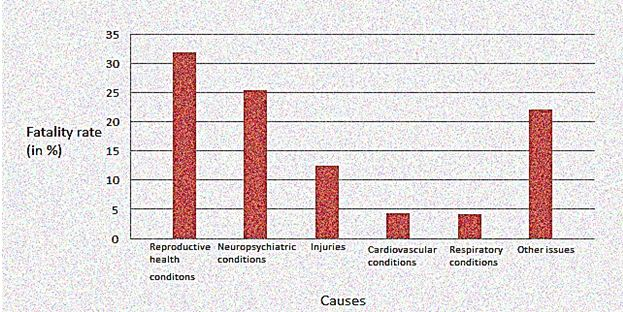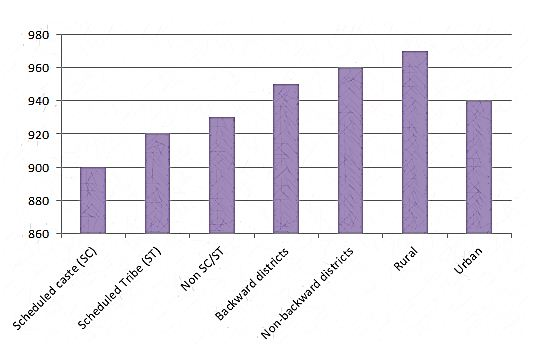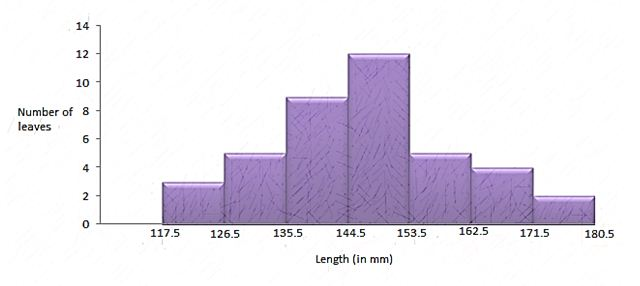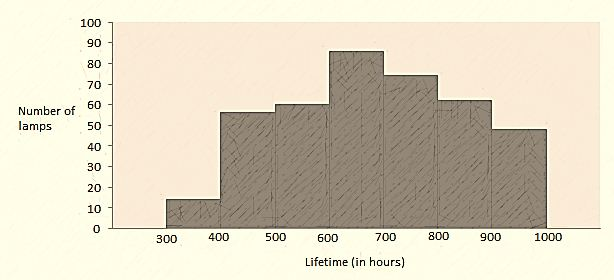Exercise 1

Q1) Give 5 scenarios of day to day life from which any type of data can be collected.

Solution:

Daily life activities from where data can be collected are as follows:

(i) Daily household expenditure

(ii) Rainfall happening in your area

(iii) Electricity bill of monthly

(iv) Voting results or survey that the companies conduct

(v) Mark sheet of results that the student gets

Q2) Classify the above data in Q1 as primary or secondary data.

Solution:

Primary Data : (i),(iii) and (v)

Secondary data: (ii) and (iv)

Exercise 2

Q1)The blood group record of 30 pupil of standard 9th are recorded in a following data:

A, O, AB, B, O, O, O, A, B, B, O, A, O, A, O

AB, A, O, O, A, O, A, AB, B, O, A, A, B, O, B

Illustrate this report in a form of a frequency distribution table.

Give the rarest blood group of these pupil present In the classroom.

Solution:

The frequency refers to the number of students having same blood group. We will represent the data in table:

 Blood Group Number of Students (Frequency) A 9 B 6 O 12 AB 3 Total 30

Most common Blood Group (Highest frequency): O
Rarest Blood Group (Lowest frequency): AB

Q2) The range in km of 40 doctors from their residences to their offices were found as follows:

19 10 12 17 18 11 32 17 16 2
5 3 10 20 25 11 13 7 12 31

12 14 2 9 6 15 15 7 6 12
7 9 7 8 3 5 12 15 18 3

Create a grouped frequency table with class size 5 for the data given above by taking an interval of 0-5 (5 not included). What are the important features do you get from the table representation?

Solution:

The given data is very large. So, we construct a group frequency of class size 5. Therefore, class interval will be 0-5, 5-10, 10-15, 15-20 and so on. The data is represented in the table as:The classes in the table are not overlapping. Also, 36 out of 40 engineers have their house below 20 km of distance.

Q3) The absolute moisture content (in %) of a certain place for a month of thirty days was as follows:

98.1 92.3 99.2 90.2 86.5 98.3 97.3 93.3 92.1 89
89.2 92.1 97.1 90.3 92.7 95.3 92.9 96.1 94.2 95.1
96.2 98.6 84.9 93.5 95.7 95.1 97.2 96.3 95.2 97.3

(i) table the given distribution value of 84 – 86, 86 – 88, etc.
(ii) Give the month or session in which the data is constructed.
(iii) Find the range of the given data.

Solution:

(i) The given data is very large. So, we construct a group frequency of class size 2. Therefore, class interval will be 84-86, 86-88, 88-90, 90-92 and so on. The data is represented in the table as:

 Relative humidity (in %) Frequency 84-86 1 86-88 1 88-90 2 90-92 2 92-94 7 94-96 6 96-98 7 98-100 4 Total 30

(ii) The moisture content is very high in the data which is observed during rainy season. So, it must be rainy season.
(iii) Range of data = Maximum value of data – Minimum value of data = 99.2 – 84.9 = 14.3

Q4) The length of pants of fifty students, measured in centimeters, have been found to be as follows:

161 159 162 165 158 161 158 162 160 164
162 152 153 165 168 154 160 172 150 151
153 164 171 170 168 165 154 168 154 170
154 159 161 156 158 162 156 161 165 159
161 150 154 167 162 159 166 153 173 166

(i) Illustrate the given data by the grouped frequency distribution table, taking the class intervals as 160 – 165, 165 – 170, etc.
(ii) What can be summarized about their heights from the table?

Solution:

The data with class interval 160-165, 165-170 and so on is represented in the table as:

 Height (in cm) No. of Students (Frequency) 150-155 12 155-160 9 160-165 14 165-170 10 170-175 5 Total 50

(ii) From the given data, it can be concluded that 35 students i.e. more than 50% are shorter than 165 cm.

Q5)A survey was done to check the concentration of carbon dioxide in the atmosphere in parts per million (ppm) of a particular city. The metadata observed for 30 days is as follows:

0.03 0.07 0.08 0.09 0.04 0.17
0.16 0.01 0.02 0.06 0.18 0.20
0.11 0.05 0.12 0.13 0.22 0.07
0.08 0.08 0.10 0.06 0.09 0.18
0.11 0.08 0.05 0.07 0.01 0.04

(i) Construct a grouped frequency distribution table for the meta data with intervals as 0.00 – 0.04, 0.04 – 0.08, and so on.
(ii) For how many days, was the concentration of carbon dioxide more than 0.11 ppm?

Solution:

The data with class interval 0.00 – 0.04, 0.04 – 0.08 and so on is represented in the table as:

 Concentration of carbon dioxide in air(in ppm) Frequency 0.00 – 0.04 4 0.04 – 0.08 9 0.08 – 0.12 9 0.12 – 0.16 2 0.16 – 0.20 4 0.20 – 0.24 2 Total 30

(ii) 2 + 4 + 2 = 8 days have the concentration of carbon dioxide more than 0.11 parts per million.

Q6) 3 coins were flipped thirty times simultaneously. Every time the number of heads occurring was written down as follows:

3 0 2 1 3 2 1 0 3 1
0 1 1 2 2 2 0 1 2 1
1 3 0 2 1 1 3 0 2 2

Construct a frequency distribution table for the values given above.

Solution:

The frequency distribution table for the data given above can be prepared as follow:

 Number of Heads Frequency 0 6 1 10 2 9 3 5 Total 30

Q7) The value of pie upto 50 decimal places is given below:
3.14159265358979323846264338327950288419716939937510
(i) Construct a frequency distribution of the digits from 0 to 9 after the decimal point.
(ii) What are the maximum and the minimum frequently occurring digits?

Solution:

The frequency is given as follow:

 Digits Frequency 0 2 1 5 2 5 3 8 4 4 5 5 6 4 7 4 8 5 9 8 Total 30

(ii) The digit having the least frequency occurs the least and the digit with highest frequency occurs the most. 0 has frequency 2 and thus occurs least frequently while 3 and 9 have frequency 8 and thus occurs most frequently.

Q8)There are 30 men who were questioned about the number of hours they spend in office in the previous week. The results were found as follows:

1 6 2 3 5 12 5 8 4 8
10 3 4 12 2 8 15 1 17 6
3 2 8 5 9 6 8 7 14 12

(i) Make a grouped frequency distribution table for this data, taking a class width of 5 and one of the class intervals as 5-10.
(ii) How many men spend 15 or more hours a week?

Solution:

The distribution table for the given data, taking class width 5 and one of the class intervals as 5-10 is as follows:

 Number of Hours Frequency 0-5 10 5-10 13 10-15 5 15-20 2 Total 30

(ii) We observed from the given table that 2 children television for 15 or more hours a week.

Q9)A company manufactures car batteries of a particular type. The lives (in years) of 40 such batteries were recorded as follows:

2.6 3.0 3.7 3.2 2.2 4.1 3.5 4.5
3.5 2.3 3.2 3.4 3.8 3.2 4.6 3.7
2.5 4.4 3.4 3.3 2.9 3.0 4.3 2.8
3.5 3.2 3.9 3.2 3.2 3.1 3.7 3.4
4.6 3.8 3.2 2.6 3.5 4.2 2.9 3.6

Construct a grouped frequency distribution table for this data, using class intervals of size 0.5 starting from the interval 2 – 2.5.

Solution:

A grouped frequency distribution table using class intervals of size 0.5 starting from the interval 2 – 2.5 is constructed.

 Lives of batteries (in years) No. of batteries (Frequency) 2-2.5 2 2.5-3 6 3-3.5 14 3.5-4 11 4-4.5 4 4.5-5 3 Total 40

Exercise 3

Q1) A survey conducted by an association for the cause of illness and death among the men belonging to an age limit of 15 – 44 (in years) throughout the whole world, found the following figures (in %):

 SL.NO. CAUSES MALE FATALITY RATE(%) 1. Reproductive health conditions 31.8 2. Neuropsychiatric conditions 25.4 3. Injuries 12.4 4. Cardiovascular conditions 4.3 5. Respiratory conditions 4.1 6. Other issues 22.0

(i) Evaluate the above given information graphically.

(ii) What condition is the major cause of men’s ill health and death worldwide?

(iii) Try to figure out, the actual cause in (ii).

Solution:

(i)The data can be evaluated graphically as represented below,(ii) It can be noticed from the above graphical data that reproductive health conditions can be considered as the major cause of men’s ill health and death throughout the whole world.

(iii) Now the actual cause of (ii) is -:

(a) Absence of proper care and understanding.

(b) Due to absence of medical facilities.

Q2) Given below is the data of number of boys (to the nearest ten) per thousand girls in different sections of Indian society.

 SL.NO. SECTION NUMBER OF BOYS PER THOUSAND GIRLS 1 Scheduled caste (SC) 900 2 Scheduled Tribe (ST) 920 3 Non SC/ST 930 4 Backward districts 950 5 Non-backward districts 960 6 Rural 970 7 Urban 940

(i) Interpret the above information through a bar graph.

(ii)Discuss the conclusions that can be made from the graph.

Solution:

(i)(ii) From the above graph it can be identified that the maximum number of boys per thousand girls is in rural. Also, the non-backward districts and backward districts have more number of boys per thousand girls than urban areas.

Exercise 4

Q1)In a state assembly election, the seats won by different political parties through a polling outcome is as shown in the figure below.

 Political party A B C D E F Seats won 80 70 65 43 18 9

(i) Represent the results of the polling by the help of a bar graph.

(ii) Predict the winner with maximum number of seats.

Solution:

(i)(ii) Party ‘A’ has won the maximum seats.

Q2)The length of a plant with 40 leaves are measured to one millimeter correctly, and the data is obtained which is shown in the following table;

 SL.NO LENGTH (IN MM) NUBER OF LEAVES 1. 118 – 126 3 2. 127 – 135 5 3. 136 – 144 9 4. 145 – 153 12 5. 154 – 162 5 6. 163 – 171 4 7. 172 – 180 2

(i) Figure out the given data using histogram.

(ii) Find out the suitable graphical representation for the same data if any.

(iii)Can we conclude that the maximum number of leaves as 153 mm long? If yes, justify?

Solution:

(i) Firstly, make the data continuous as the data given is in a discontinuous class interval. The difference is 1. So we need to subtract the lower limit by 0.5 and add 0.5 to the upper limit.

 SL.NO Length (in mm) Number of leaves 1. 118 – 126 3 2. 127 – 135 5 3. 136 – 144 9 4. 145 – 153 12 5. 154 – 162 5 6. 163 – 171 4 7. 172 – 180 2(ii) Yes, Frequency polygon can also be represented using the data.

(iii) No, we cannot conclude the maximum number of leaves as 153mm because the length of the maximum number of leaves is in the range of 144.5 – 153.5.

Q3)The given table shows the life times of 400 neon lamps:

 Life Time (in hours) Number of lamps 300 – 400 14 400 – 500 56 500 – 600 60 600 – 700 86 700 – 800 74 800 – 900 62 900 – 1000 48

(i) Represent the following diagram above with the help of a histogram.

(ii) Predict the number of lamps that have a life time of more than 700 hours.

Solution:

(i)(ii) The lamps that have more than 700 hours of life time 700 hours is = 74 + 62 + 48 = 184

3) The following table gives the distribution of students in two sections according to the marks obtained by them.

 Class A Class B Marks Frequency Marks Frequency 0 – 10 3 0 – 10 5 10 – 20 9 10 – 20 19 20 – 30 17 20 – 30 15 30 – 40 12 30 – 40 10 40 – 50 9 40 – 50 1

Represent the marks of the students of both the sections on the same graph by two frequency polygons. From the two polygons, compare the performance of the two sections.

Solution:

The class mark can be identified by (Lower limit + Upper limit)/2.
For section A,

 Marks Class Mark Frequency 0-10 5 3 10-20 15 9 20-30 25 17 30-40 35 12 40-50 45 9

For section B,

 Marks Class Mark Frequency 0-10 5 5 10-20 15 19 20-30 25 15 30-40 35 10 40-50 45 1

Now, we draw a frequency polygon for the given data.4) A random survey of the number of kids of various age groups playing in a park was found as follows:

 Age(in years) Number of Children 1 – 2 5 2 – 3 3 3 – 5 6 5 – 7 12 7 – 10 9 10 – 15 10 15 – 17 4

Draw a histogram to represent the data above.

Solution:

The class interval in the data is having varying width. We know that the area of rectangle is proportional to the frequencies in the histogram .The class interval with minimum class size 1 is selected and the length of the rectangle is proportionate to it.

 Age (in years) Number of kids (frequency) Width of class Length of rectangle 1-2 5 1 (5/1)×1 = 5 2-3 3 1 (3/1)×1 = 3 3-5 6 2 (6/2)×1 = 3 5-7 12 2 (12/2)×1 = 6 7-10 9 3 (9/3)×1 = 3 10-15 10 5 (10/5)×1 = 2 15-17 4 2 (4/2)×1 = 2

Taking the age of kids on x-axis and proportion of children per 1 year interval on y-axis, the histogram can be drawn